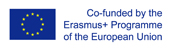This project has been funded with support from the European Commission.
This web site reflects the views only of the author, and the Commission cannot be held responsible for any use which may be made of the information contained therein.

Select language   >   IT EN RO PL LT

### Pythagorean theorem

Subject taught
- Math

Type of Product
- Exercises

Language Skills Developed
- Listening
- Speaking
- Writing

Transferable/Scientific Skills Developed
Student will develop self-motivation, analytical skills, problem-solving skills.

Description
General aims
Student will be able to use Pythagorean theorem to solve different types of word problems using the Pythagorean Theorem.

Student will know basic vocabulary in English connected with geometry. Student will develop positive learning habits such as organisational and problem-solving skills.

Linguistic aims:
- to read English with ease
- to understanding content
- to learn from written texts
- to master tools for gathering information
- to express understanding and experiences
- to enable students to understand topic correctly
- to translate words connected with Pythagoras theorem in English into the mother tongue using one’s own words.

Subject specific aims:
- student is able to use Pythagoras theorem in math problems which are connected directly with a right triangle
- student gains confidence in their math skills
- student is able to solve basic problems connected with Pythagoras theorem, which can be used in the real life
- student has unlimited access to free educational content one can master at their own pace
- student is better prepared for standardized testing.

Target group age:
Students 14-15 years old, before using the resource they need to know how to do basic operation with powers, roots, solve equations. Students also have to be familiarized with Pythagoras theorem. What is more, these exercises can be used by anyone who wants to practice Pythagoras theorem, also by grade teachers who wants to teach math bilingually.

Level of competence in English (CEFR)
B1/B1+

Time required to use the resource with the students:
the teacher can use the resource with the students for one lesson.

How to use it
Before using the exercises during one’s classes, the students should be able to do basic operation with powers, roots, equations and know Pythagoras theorem.

During lessons the teacher should ask the student some questions in English, for example:
“Which side of right angle is called hypotenuse? ” “How can I tell which side is hypotenuse?”
“What is the Pythagorean Theorem in your own words?”
“How to solve hypotenuse?”

The students give the answers with the examples. The teacher should give them feedback and show students more examples on the black table. The teacher highlights some new words for students in English and students should find the translation these words into the mother tongue and write down the new words in English in the notebook with the right translation in their own words. The words which should be taken into consideration are: right angle, right triangle, hypotenuse, square root.
The teacher gives examples how to use Pythagorean theorem to find right triangle side lengths. Students practice it in small groups.
The teacher asks students to do the exercises of Pythagoras' Theorem in pairs from the resource and gives students opportunity to make notes, asks questions and develop some problems on the black table. The teacher gives a feedback to the students and helps them determine the Pythagoras theorem of given examples if they need it.
Possible difficulties for the students
Students who have poor knowledge of English can have problems with understanding the content. Students who has problems doing basic geometry and operations with powers and roots or solving equations can face difficulties in solving math problems with the Pythagorean theorem which are introduced in the resource which is recommended.